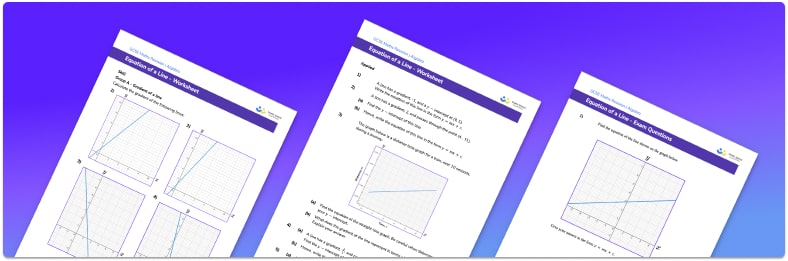# Equation Of A Line Worksheet• Section 1 of the equation of a line worksheet contains 20+ skills-based equation of a line questions, in 3 groups to support differentiation
• Section 2 contains 3 applied equation of a line questions with a mix of worded problems and deeper problem solving questions
• Section 3 contains 3 foundation and higher level GCSE exam style questions on equation of a line
• Answers and a mark scheme for all equation of a line questions are provided
• Questions follow variation theory with plenty of opportunities for students to work independently at their own level
• All questions created by fully qualified expert secondary maths teachers
• Suitable for GCSE maths revision for AQA, OCR and Edexcel exam boards

• This field is for validation purposes and should be left unchanged.

You can unsubscribe at any time (each email we send will contain an easy way to unsubscribe). To find out more about how we use your data, see our privacy policy.

### Equation of a line at a glance

Each straight line has an equation. Horizontal lines have equations of the form y=a and vertical lines have equations of the form x=a, where a is a constant. Sloping lines have equations of the form y=mx+c where m is the gradient of the line and c is the y intercept of the line.

To find the equation of a straight line, we start by finding the gradient of the line. To do this, we pick two points on the line and calculate (change in y) / (change in x) between the two points. This tells us the gradient, how steep the line is. We then need to find the y intercept. The y intercept is the y-coordinate of the point at which the line crosses the y axis. We can then insert the gradient, m, and the y intercept, c, into the equation y=mx+c. For example, if the gradient of a line is 2 and it crosses the y axis at (0, 1), the equation of the line would be y=2x+1.

Looking forward, students can then progress to additional straight line graphs worksheets and other algebra worksheets, for example a sequences worksheet, simultaneous equations worksheet or straight line graphs worksheet.For more teaching and learning support on Algebra our GCSE maths lessons provide step by step support for all GCSE maths concepts.

## Do you have KS4 students who need more focused attention to succeed at GCSE?There will be students in your class who require individual attention to help them succeed in their maths GCSEs. In a class of 30, it’s not always easy to provide.

Help your students feel confident with exam-style questions and the strategies they’ll need to answer them correctly with our dedicated GCSE maths revision programme.

Lessons are selected to provide support where each student needs it most, and specially-trained GCSE maths tutors adapt the pitch and pace of each lesson. This ensures a personalised revision programme that raises grades and boosts confidence.

Find out more